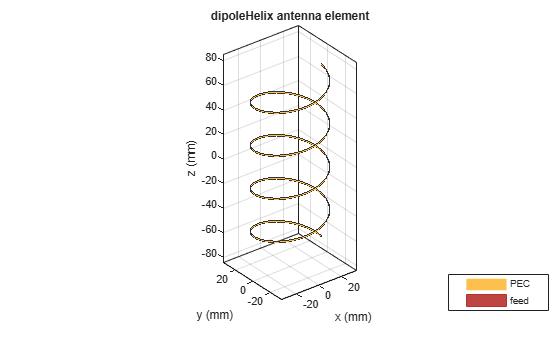dipoleHelix

Create helical dipole antenna

Description

The dipoleHelix object is a helical dipole antenna. The antenna is typically center-fed. You can move the feed along the antenna length using the feed offset property. Helical dipoles are used in satellite communications and wireless power transfers.

The width of the strip is related to the diameter of an equivalent cylinder by this equation

$w=2d=4r$

where:

• w is the width of the strip.

• d is the diameter of an equivalent cylinder.

• r is the radius of an equivalent cylinder.

For a given cylinder radius, use the cylinder2strip utility function to calculate the equivalent width. The default helical dipole antenna is center-fed. The circular ground plane is on the xy- plane. Commonly, helical dipole antennas are used in axial mode. In this mode, the helical dipole circumference is comparable to the operating wavelength, and has maximum directivity along its axis. In normal mode, the helical dipole radius is small compared to the operating wavelength. In this mode, the helical dipole radiates broadside, that is, in the plane perpendicular to its axis. The basic equation for the helical dipole antenna is:

$\begin{array}{l}x=r\mathrm{cos}\left(\theta \right)\\ y=r\mathrm{sin}\left(\theta \right)\\ z=S\theta \end{array}$

where:

• r is the radius of the helical dipole.

• θ is the winding angle.

• S is the spacing between turns.

For a given pitch angle in degrees, use the helixpitch2spacing utility function to calculate the spacing between the turns in meters.Creation

Description

example

dh = dipoleHelix creates a helical dipole antenna. The default antenna operates around 2 GHz.

example

dh = dipoleHelix(Name,Value) creates a helical dipole antenna, with additional properties specified by one or more name–value pair arguments. Name is the property name and Value is the corresponding value. You can specify several name-value pair arguments in any order as Name1, Value1, ..., NameN, ValueN. Properties not specified retain their default values.

Properties

expand all

Turn radius, specified as a scalar in meters.

Data Types: double

Strip width, specified as a scalar in meters.

Note

Strip width should be less than 'Radius'/5 and greater than 'Radius'/250. 

Example: 'Width',5

Data Types: double

Number of turns of the helical dipole, specified a scalar.

Example: 'Turns',2

Data Types: double

Spacing between turns, specified as a scalar in meters.

Example: 'Spacing',1.5

Data Types: double

Direction of helical dipole turns (windings), specified as 'CW' or 'CCW'.

Example: 'WindingDirection','CW'

Data Types: char | string

Type of the metal used as a conductor, specified as a metal material object. You can choose any metal from the MetalCatalog or specify a metal of your choice. For more information, see metal. For more information on metal conductor meshing, see Meshing.

Example: m = metal('Copper'); 'Conductor',m

Example: m = metal('Copper'); ant.Conductor = m

Lumped elements added to the antenna feed, specified as a lumped element object. For more information, see lumpedElement.

Example: 'Load',lumpedelement. lumpedelement is the object for the load created using lumpedElement.

Signed distance from center along length and width of ground plane, specified as a two-element vector in meters. Use this property to adjust the location of the feedpoint relative to the ground plane and patch.

Example: 'FeedOffset',[0.01 0.01]

Data Types: double

Tilt angle of the antenna, specified as a scalar or vector with each element unit in degrees. For more information, see Rotate Antennas and Arrays.

Example: 'Tilt',90

Example: ant.Tilt = 90

Example: 'Tilt',[90 90],'TiltAxis',[0 1 0;0 1 1] tilts the antenna at 90 degrees about the two axes defined by the vectors.

Note

The wireStack antenna object only accepts the dot method to change its properties.

Data Types: double

Tilt axis of the antenna, specified as:

• Three-element vector of Cartesian coordinates in meters. In this case, each coordinate in the vector starts at the origin and lies along the specified points on the X-, Y-, and Z-axes.

• Two points in space, each specified as three-element vectors of Cartesian coordinates. In this case, the antenna rotates around the line joining the two points in space.

• A string input describing simple rotations around one of the principal axes, 'X', 'Y', or 'Z'.

Example: 'TiltAxis',[0 1 0]

Example: 'TiltAxis',[0 0 0;0 1 0]

Example: ant.TiltAxis = 'Z'

Note

The wireStack antenna object only accepts the dot method to change its properties.

Data Types: double

Object Functions

 show Display antenna or array structure; display shape as filled patch info Display information about antenna or array axialRatio Axial ratio of antenna beamwidth Beamwidth of antenna charge Charge distribution on metal or dielectric antenna or array surface current Current distribution on metal or dielectric antenna or array surface design Design prototype antenna or arrays for resonance around specified frequency efficiency Radiation efficiency of antenna EHfields Electric and magnetic fields of antennas; Embedded electric and magnetic fields of antenna element in arrays impedance Input impedance of antenna; scan impedance of array mesh Mesh properties of metal or dielectric antenna or array structure meshconfig Change mesh mode of antenna structure optimize Optimize antenna or array using SADEA optimizer pattern Radiation pattern and phase of antenna or array; Embedded pattern of antenna element in array patternAzimuth Azimuth pattern of antenna or array patternElevation Elevation pattern of antenna or array rcs Calculate and plot radar cross section (RCS) of platform, antenna, or array returnLoss Return loss of antenna; scan return loss of array sparameters Calculate S-parameter for antenna and antenna array objects vswr Voltage standing wave ratio of antenna

Examples

collapse all

Create a default helical dipole antenna and view it.

dh = dipoleHelix
dh =
dipoleHelix with properties:

Width: 1.0000e-03
Turns: 3
Spacing: 0.0350
WindingDirection: 'CCW'
FeedOffset: 0
Conductor: [1x1 metal]
Tilt: 0
TiltAxis: [1 0 0]

show(dh)Create a four-turn helical dipole antenna with a turn radius of 28 mm and a strip width of 1.2 mm.

show(dh)Plot the radiation pattern of the helical dipole at 1.8 GHz.

pattern(dh, 1.8e9);Balanis, C. A. Antenna Theory. Analysis and Design. 3rd Ed. Hoboken, NJ: John Wiley & Sons, 2005.

 Volakis, John. Antenna Engineering Handbook. 4th Ed. New York: McGraw-Hill, 2007.